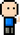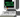# Josh Meets Computer# Returning minimum coins for change

June 19, 2018

This note is around ultimately developing a dynamic programming algorithm while exploring other approaches to solving a coin change challenge. The challenge is that you are building an international vending machine and need to write code to return the proper amount of change. The following shows a few approaches on implementing an algorithm to return the least amount of coins needed to return change.

### First Approach - Greedy Method

This method is probably the most intuitive approach. The algorithm starts with the largest coin and tries to solve the problem (return exact change) the best it can with this coin until it can’t, only then does it move to the next smaller coin. It continues to compare and subtract the coin value to change left (next coin if too small of change), until there is no change left.

``````def greedy_change(change, coins):

coins = sorted(coins, reverse=True)
num_coins = 0
type_coins = []

for c in coins:
while change >= c:
num_coins += 1
type_coins += [c]

change -= c

if change == 0:
break

return num_coins, type_coins

change = 37
coins = [1, 5, 10, 21, 25]
print('We need {1} coins to produce enough change for {0} cents: {2}'.format(change, *greedy_change(change, coins)))``````
``We need 4 coins to produce enough change for 37 cents: [25, 10, 1, 1]``

This method doesn’t work well when there is a lower value coin that divides perfectly into the change. For example, what happens when we need to return 63 cents and there is a 21 cent coin in our collection? The minimum number of coins should be 3 21 cent coins:

``````change = 63
coins = [1, 5, 10, 21, 25]
print('Available coins: {}\n'.format(coins))
print('We need {1} coins to produce enough change for {0} cents: {2}'.format(change, *greedy_change(change, coins)))``````
``````Available coins: [1, 5, 10, 21, 25]

We need 6 coins to produce enough change for 63 cents: [25, 25, 10, 1, 1, 1]``````

### Second Approach - Solving with Recursion

The next method will use recursion. Algorithms with recursion start with a base case (the bottom of the recursion) that returns a value, followed by a routine the calls the function itself with a new set of values. The function recurs until it reaches the base case, then returns the value for each time the function was called (in reverse order).

The idea with this algorithm is we want the minimum change to be either:

• A penny, plus the minimum change required for the remainder (change minus a penny)
• A nickle, plus the minimum change required for the remainder (change minus a nickle)
• A dime, plus the minimum change required for the remainder (change minus a dime)
• A quarter, plus the minimum change required for the remainder (change minus a quarter)

From these options, we return whichever one of these results in the minimum number of coins. We have to recursively call the function itself to determine “the minimum change required for the remainder” part.

``````def recur_change(change, coins):

# base case: make exact change (only one more coin needed)
if change in coins:
return 1
else:
eligible_coins = [c for c in coins if c < change]

for c in eligible_coins:
remainder = change - c
num_coins = 1 + return_change(remainder, eligible_coins)

if num_coins < min_coins:
min_coins = num_coins

return min_coins``````
``print('We need {1} coins for {0} cents in change.'.format(change, recur_change(change, coins)))``
``We need 3 coins for 63 cents in change.``

While this answer is now correct, it’s extremely inefficient. The function makes an incredible amount of function calls to explore all coin combinations and find the minimum number of coins to return for each remaining change, even though the solution set is much smaller. This is because it recomputes sub-problems that have already been solved in earlier recursions. For example, figuring out the remaining change for 15 cents takes 52 recursive function calls, and there are three instances.

Looking at the timed run below, it took a whopping 50 seconds to figure out the change! Who wants to stand at a vending machine that long?

``%timeit -n 1 -r 1 recur_change(change, coins)``
``50.3 s ± 0 ns per loop (mean ± std. dev. of 1 run, 1 loop each)``

### Third Approach - Memoization (Caching)

A third approach is to remember the results as we calculate them. This would prevent the extra recursive function calls to recalculate minimum change for certain values previously solved for.

``````def cache_change(change, coins, known):

min_coins = change

if change in coins:
known[change] = 1
return 1, known

elif known[change] > 0:
return known[change], known

else:
eligible_coins = [c for c in coins if c <= change]

for c in eligible_coins:
remainder = change - c
num_coins = 1 + cache_change(remainder, eligible_coins, known)

if num_coins < min_coins:
min_coins = num_coins
known[change] = min_coins
return min_coins, known
``````
``````known_coins =  * (change + 1)  # start with 0 for known amount of change required for each possible change amount
num_coins, cached_values = cache_change(63, [1,5,10,21, 25], known_coins)
print('We need {} coins for {} cents in change.'.format(num_coins, change))``````
``We need 3 coins for 63 cents in change.``
``%timeit -n 1 cache_change(63, [1,5,10,21, 25], known_coins)``
``````The slowest run took 7.00 times longer than the fastest. This could mean that an intermediate result is being cached.
1.93 µs ± 1.22 µs per loop (mean ± std. dev. of 7 runs, 1 loop each)``````

### Fourth Approach - Dynamic Programming

A systematic approach would be to calculate the minimum number of coins needed to produce change for any amount of value, starting from one cent and working our way up to the desired value. The first loop starts with 1 cent and determines the number of coins needed to make change for one cent. It does this by first declaring the number of pennies to make the correct change.

From there, the algorithm goes through each eligible coin and subtracts the coins value from the change. With this remaining amount, it checks the coin table for how many coins are needed plus a coin (because we had just used a coin to subtract value from the change amount).

If this value is less than the current amount of coins needed (which starts with the number of pennies needed to make change), it a new value to the amount of coins needed.

After going through all the eligible coins, whichever iteration produced the lowest number of coins gets assigned to the specific cent value in our coin table. The algorithm then goes to the next incremental cent.

At the end, we just pick the value from the table corresponding to our change, which was the last cent value calculated.

``````def dynamic_change(change, coins, known):
coins_used =  * (change + 1)

for cents in range(change + 1):
coins_needed = cents  # starting with <cents> number of pennies
new_coin = 1
eligible_coins = [c for c in coins if c <= cents]

for coin in eligible_coins:
if known[cents - coin] + 1 < coins_needed:
coins_needed = known[cents - coin] + 1  # plus one because we used a coin subtracting coin from cents
new_coin = coin
known[cents] = coins_needed
coins_used[cents] = new_coin

return known[change], coins_used

def print_coins(coins_used, change):
coin = change
result = []

while coin > 0:
thisCoin = coins_used[change]
result += [thisCoin]
coin -= thisCoin

return result``````
``````known_coins =  * (change + 1)  # start with 0 for known amount of change required for each possible change amount
num_coins, coins_used = dynamic_change(63, [1,5,10,21, 25], known_coins)
print('We need {} coins for {} cents in change, {}'.format(num_coins, change, print_coins(coins_used, change)))``````
``We need 3 coins for 63 cents in change, [21, 21, 21]``
``%timeit -n 1 dynamic_change(63, [1,5,10,21, 25], known_coins)``
``147 µs ± 58 µs per loop (mean ± std. dev. of 7 runs, 1 loop each)``